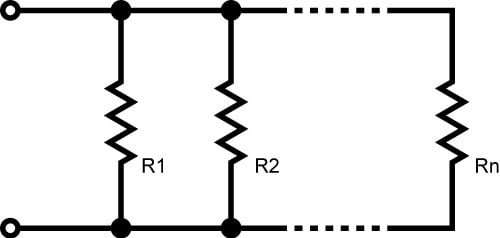# Parallel Resistance Calculator

## Calculate the total resistance of resistors in parallel with ease!

(Ω)

(Ω)

### How to Calculate the Total Resistance of Resistors in ParallelCalculating the equivalent resistance (REQ) of resistors in parallel by hand can be tiresome. This tool was designed to help you quickly calculate equivalent resistance of up to 6 resistors connected in parallel. To use it, just specify how many parallel resistors there are and the resistance value for each one. If you have more than 6 resistors, simply use the calculator to determine the equivalent resistance of the first 6 resistors, and then plug that value in for R1 and add values for R7, R8, ..., R11 into the calculator in the R2, R3, ..., R6 input fields.

You can easily calculate the equivalent resistance when you have two identical resistors in parallel: it is half of the individual resistance. This is handy when you need a specific resistance value and don't have an appropriate part readily available. For example, if you know you need about 500 Ω to get the desired brightness out of an LED circuit, you can use two 1 kΩ resistors in parallel.

Keep in mind that the current through an individual resistor does not change when you add resistors in parallel, because adding resistors in parallel does not affect the voltage across the resistors' terminals. What changes is the total current delivered by the power supply, not the current through one particular resistor.

### Equations

$$\frac{1}{R_{EQ}} = \frac{1}{R_{1}} + \frac{1}{R_{2}} + \frac{1}{R_{3}} + ... + \frac{1}{R_{N}}$$

When you have only two resistors in parallel: $$R_{EQ} = \frac{R_1\times R_2}{R_1+R_2}$$

### Applications

Resistors in series are equivalent to one resistor whose resistance is the sum of each individual resistor. Resistors in parallel, on the other hand, result in an equivalent resistance that is always lower than every individual resistor. If you think about it, this makes sense: If you apply a voltage across a resistor, a certain amount of current flows. If you add another resistor in parallel with the first one, you have essentially opened up a new channel through which more current can flow. No matter how large the second resistor is, the total current flowing from the power supply will be at least slightly higher than the current through the single resistor. And if the total current is higher, the overall resistance must be lower.• M
mmikerosss September 15, 2017

Great tool, but there seems to be an error! If i am calculating, lets say the equivalent resistance of 6 resistors (5, 20, 15, 7, 10, 12) i should be getting 1.56ohms but instead i get 2.26ohms. Please try to fix the algorithm, otherwise great tool!

Like.
• R
Robert Keim September 21, 2017
I tried it with the same resistances and got 1.56 Ω, as expected. Are you still seeing 2.26 Ω?
Like.
•mrrobin November 15, 2017
I agree with you. I got same value.
Like.